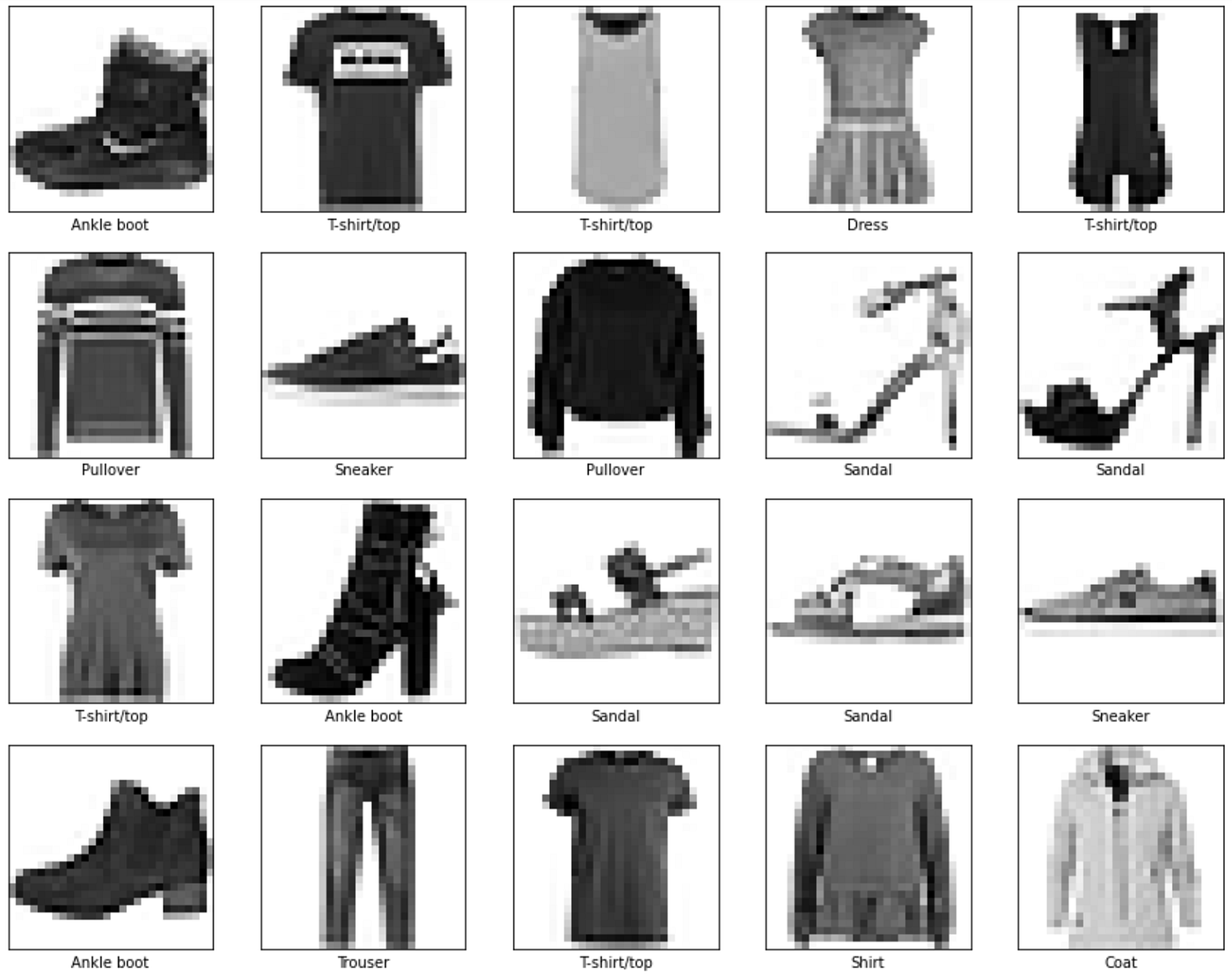# Solving the Fashion MNIST with a simple neural network

## Fashion MNIST datasetThe first 20 photos and labels of the Fashion MNIST training set.
1. Dense neural networks
2. Convolutional neural networks

## Coding the neural network

`%tensorflow_version 2.ximport tensorflow as tffrom tensorflow import kerasimport numpy as npimport matplotlib.pyplot as plt`
`# load the fashion mnist dataset from kerasfashion_mnist = keras.datasets.fashion_mnist (train_X, train_y),(test_X,test_y) = fashion_mnist.load_data()`
`# data preprocessing, scaling values from 0-255 to 0-1train_X = train_X/255.0test_X = test_X/255.0`
`model = tf.keras.models.Sequential([   tf.keras.layers.Flatten(input_shape=(28, 28)),   tf.keras.layers.Dense(128, activation='relu'),      tf.keras.layers.Dense(10, activation='softmax')     ])`
`model.compile(optimizer='adam',              loss=tf.keras.losses.SparseCategoricalCrossentropy(from_logits=True),              metrics=['accuracy'])`
`model.fit(train_X, train_y, epochs=12)`
`loss, acc = model.evaluate(test_X, test_y, verbose = 1)print('\\nTest accuracy: ', acc)`

--

--